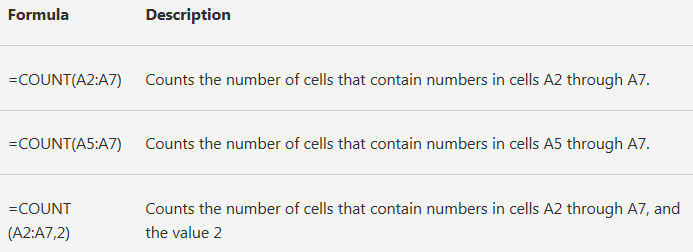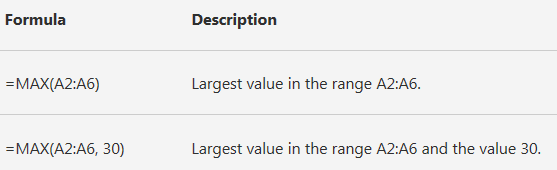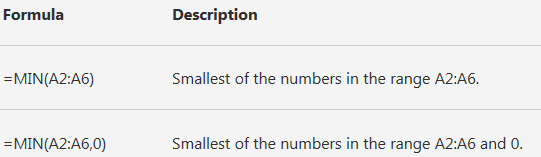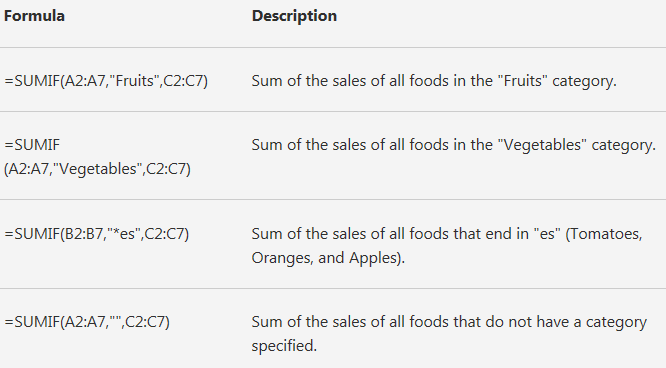# OzGrid

Lesson 22 - Excel's Useful Functions

Category: [Excel]  Demo Available

# EXCEL'S USEFUL FUNCTIONS

## Lesson 22 - Using Some of Excel's most Common Functions

### USEFUL FUNCTIONS

Now we have covered the SUM function in detail and also covered formula arguments and formula syntax we will use half of this lesson to look at some of Excels easy to use Functions. Although these functions are among the easiest to use they are also arguably the most useful.

Once we have covered these functions we will go into some detail on Excels Insert Function. The Insert Function was known as the Paste Function in earlier versions of Excel. The Insert Function houses all of Excel’s built-in functions under their appropriate categories and goes a long way to writing the chosen function for us. In case you are wondering why we don’t just skip all the detail and go straight to the Insert Function and make life easier for us all, it is because I firmly believe that the most important aspects of Excel and it’s functions and formulas is understanding them. I like to believe that by course completion I will have taught you Excel, not shown it to you!

The functions we shall look at first are:

• AVERAGE
• COUNT
• COUNTA
• COUNTBLANK
• COUNTIF
• MAX
• MIN
• SUMIF

We will Start each description with what the function does, followed by its syntax and then the number of arguments it can accept. It is important to note that while some functions take more than one argument it is not always the case that they must all be supplied.

These arguments are known as option arguments and I will identify these in the syntax description by not bolding the argument. For example the SUM function can take up to 30 arguments, but only one of the thirty needs to be supplied, so I would show this as below, eg:

Syntax SUM(number1,number2,. . . ) Meaning “number2,…. to number 30” are all optional arguments while “number1” must be supplied.

AVERAGE

The AVERAGE function is used to return the average of the arguments supplied.

Syntax

AVERAGE(number1,number2, . . . )

The AVERAGE function can take up to 30 arguments.

The arguments supplied must be numeric or references to numeric values. Text and/or references to text are ignored. It is important to note that cells containing zeros are NOT ignored. This can give you unexpected results if you are not aware of it.

=AVERAGE(A1:A3) would equal 10 if A1:A3 contained 5, 10, 15 respectively

COUNT

The COUNT function is used to count numbers or references to numbers in a range.

Syntax

COUNT(value1,value2, . . . )

The COUNT function takes up to 30 arguments and each argument can be a variety of data types, but only numbers are counted.

If the range reference supplied contains valid dates these will also be counted.

=COUNT(A1:A5) would equal 3 if cells A1:A5 contained 10, 12/12/2001, house, 0, dog

COUNTA

The COUNTA function is used to count non-empty cells.

Syntax

COUNTA(value1,value2, . . . )

The COUNTA function takes up to 30 arguments and each argument should be a reference to a range. Cells within the range can be a variety of data types, but only non-empty cells are counted.

=COUNTA(A1:A5) would equal 4 if cells A1:A5 contained , 12/12/2001, house, 0, dog.

In other words A1 is empty and so is not counted while all other cells are.

COUNTBLANK

The COUNTBLANK function is used to count empty cells. It is the opposite of the COUNTA function

Syntax

COUNTBLANK(range)

The COUNTBLANK function takes 1 argument and this argument should be a reference to a range. Cells within the range can be a variety of data types, but only empty cells are counted

=COUNTBLANK(A1:A5) would equal 1 if cells A1:A5 contained , 12/12/2001, house, 0, dog

In other words A1 is empty and so is counted while all other cells are not.

COUNTIF

The COUNTIF function is used to count cells within a range that meet a specified criterion.

Syntax

COUNTIF(range,criteria)

The COUNTIF function takes two (2) arguments. The range argument is a reference to a range of cells, while the criteria argument is the criterion that should be met by the cells within range before they are counted. The criteria specified can be in the form of a number, text or an expression.

Number criteria

=COUNTIF(A1:A5,20) would equal 1 if cells A1:A5 contained 15, 22, 20, 0, dog

In other words A3 is the only cell that meets the criteria of 20

Text criteria

=COUNTIF(A1:A5,”dog”) would equal 1 if cells A1:A5 contained 15, 22, 20, 0, dog

In other words A5 is the only cell that meets the criteria of “dog

Expression criteria

=COUNTIF(A1:A5,”<20”) would equal 2 if cells A1:A5 contained 15, 22, 20, 0, dog.

In other words A1 and A4 are the only cells that meets the criteria of “<20”.MAX

The MAX function is used to return the largest number from a set of values.Syntax

MAX(number1,number2,. . . )

The MAX function takes up to 30 arguments and will ignore text.

=MAX(A1:A5) would equal 10 if cells A1:A5 contained 9, 8, house, 10, -10

MIN

Opposite to Max, the MIN function is used to return the smallest number from a set of values.Syntax

MIN(number1,number2,. . . )

The MIN function takes up to 30 arguments and will ignore text.

=MIN(A1:A5) would equal 1 if cells A1:A5 contained 9, 8, house, 10, 1.

SUMIF

The SUMIF function is used to return the sum value from a specified range that meets a criterion.Syntax

SUMIF(range,criteria,sum_range)

The SUMIF takes up to 3 arguments. The range is the range of cells to evaluate to see if they meet the specified criteria. The criteria specified can be in the form of a number, text or an expression. The sum_range is the range of cells to sum, but only if the corresponding cells in the range meet the specified criteria. If sum_range is omitted then the cells within the range are summed.

=SUMIF(A1:A5,5) would equal 10 if cells A1:A5 contained 5, 8, house, 10, 5

In other words cells A1 and A5 would be summed as they meet the criteria and NO sum_range was supplied.

=SUMIF(A1:A5,5,B1:B5) would equal 20 if cells A1:A5 contained 5, 8, 1, 9, 5 and cells B1:B5 contained 10,1,3,8,10.

In other words cells B1 and B5 would be summed as the corresponding cells in A1:A5 have a value of 5.

=SUMIF(A1:A5,”Cat”,B1:B5) would equal 15 if cells A1:A5 contained Cat, cat, Cat, 9, 5 and cells B1:B5 contained 5,5,5,8,11

In other words cells B1,B2 and B3 would be summed as the corresponding cells in A1:A5 contain the text “Cat” (not case sensitive).

=SUMIF(A1:A5,”>5”) would equal 34 if cells A1:A5 contained 10, 15, Cat, 9, 5 and In other words cells A1, A2 and A4 would be summed as they meet the criteria of being greater than 5.

Lots More On Excel Formulas Here

Microsoft ® and Microsoft Excel ® are registered trademarks of Microsoft Corporation. OzGrid is in no way associated with Microsoft

Go back to:

Click here to visit our Free 24/7 Excel/VBA Help Forum where there are thousands of posts you can get information from, or you can join the Forum and post your own questions.

### Gallery

stars (0 Reviews)